Test Description

## 15 Questions MCQ Test Mathematics (Maths) Class 9 | Test: Cyclic Quadrilaterals

Test: Cyclic Quadrilaterals for Class 9 2023 is part of Mathematics (Maths) Class 9 preparation. The Test: Cyclic Quadrilaterals questions and answers have been prepared according to the Class 9 exam syllabus.The Test: Cyclic Quadrilaterals MCQs are made for Class 9 2023 Exam. Find important definitions, questions, notes, meanings, examples, exercises, MCQs and online tests for Test: Cyclic Quadrilaterals below.
Solutions of Test: Cyclic Quadrilaterals questions in English are available as part of our Mathematics (Maths) Class 9 for Class 9 & Test: Cyclic Quadrilaterals solutions in Hindi for Mathematics (Maths) Class 9 course. Download more important topics, notes, lectures and mock test series for Class 9 Exam by signing up for free. Attempt Test: Cyclic Quadrilaterals | 15 questions in 15 minutes | Mock test for Class 9 preparation | Free important questions MCQ to study Mathematics (Maths) Class 9 for Class 9 Exam | Download free PDF with solutions
 1 Crore+ students have signed up on EduRev. Have you?
Test: Cyclic Quadrilaterals - Question 1

### In the given figure, ∠M is 75° then ∠O is equal to.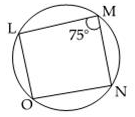Detailed Solution for Test: Cyclic Quadrilaterals - Question 1 We know that, sum of all angles of rectangle =360 so, the sum of 2 angles of rectangle = 360\2 = 180  Angle A + Angle B=180  75+B=180 or, B=180-75 or, B=105 degree.
Test: Cyclic Quadrilaterals - Question 2

### A, B, C and D are four points on a circle. AC and BD intersect at E such that angle BEC =140° and angle ECD = 25°, then angle BAC is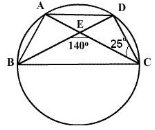Test: Cyclic Quadrilaterals - Question 3

### ABCD is a cyclic quadrilateral in which AC and BD are its diagonals. If angle DBC = 45° and angle BAC = 30°, the measure of angle BCD is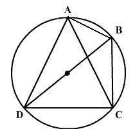Test: Cyclic Quadrilaterals - Question 4

In the figure, triangle ABC is an isosceles triangle with AB = AC and measure of angle ABC = 50°. Then the measure of angle BDC and angle BEC will be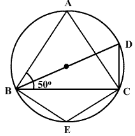Test: Cyclic Quadrilaterals - Question 5

In the figure, quadrilateral PQRS is cyclic. If ∠P = 80°, then ∠R =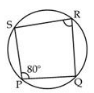Test: Cyclic Quadrilaterals - Question 6

If AB = 12 cm, BC = 16 cm and AB ⊥ BC, then radius of the circle passing through A,B and C is

Detailed Solution for Test: Cyclic Quadrilaterals - Question 6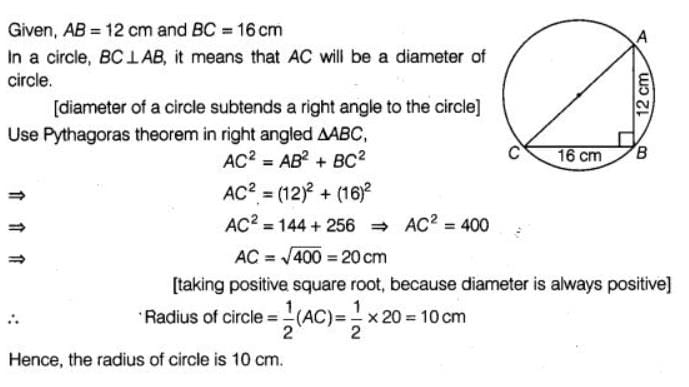Test: Cyclic Quadrilaterals - Question 7

In the figure, ABC is an equilateral triangle. Measure of angle BEC is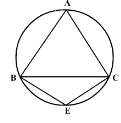Test: Cyclic Quadrilaterals - Question 8

In the figure, ∠M = 82°, then O = ?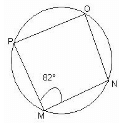Test: Cyclic Quadrilaterals - Question 9

In the figure, ABCD is a cyclic quadrilateral. AF is drawn parallel to CB and DA is produced to point E. If angle ADC = 92°, angle FAE = 20°; then angle BCD will be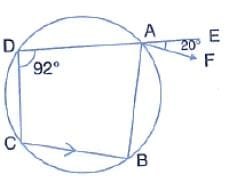Test: Cyclic Quadrilaterals - Question 10

If ∠C = ∠D = 50° then four points A, B, C, D: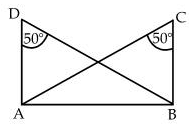Test: Cyclic Quadrilaterals - Question 11

In the figure, angle BAD = 75°, angle DCF = x and angle DEF = y.The values of x and y are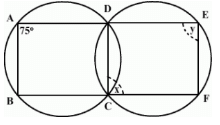Test: Cyclic Quadrilaterals - Question 12

If the sum of a pair of opposite angles of a quadrilateral is 180o, the quadrilateral is:

Test: Cyclic Quadrilaterals - Question 13

In the figure, O is the centre of the circle. The measure of angle CBD is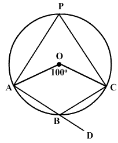Test: Cyclic Quadrilaterals - Question 14

ABCD is a cyclic quadrilateral in a circle with centre O. If angle ADC = 130°; then angle BAC is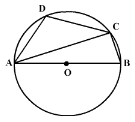Test: Cyclic Quadrilaterals - Question 15

ABC is an isosceles triangle in which AB = AC. If D and E are the mid points of AB and AC respectively, then the points D, B, C and E are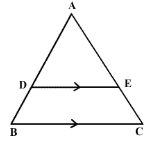## Mathematics (Maths) Class 9

88 videos|397 docs|109 tests
 Use Code STAYHOME200 and get INR 200 additional OFF Use Coupon Code